#### 期刊菜单

A Method for Determining the Evaluation Factors of Urban Tourism
DOI: 10.12677/ASS.2019.89222, PDF, HTML, XML, 下载: 505  浏览: 864

Abstract: Principal Component Analysis (PCA) is an important simplified algorithm in multivariate data analysis. SPSS is statistical analysis software. Based on the principal component analysis method, this paper uses SPSS to analyze and process the relevant data of tourism influencing factors in different cities, constructs the relevant evaluation index, and carries out cluster analysis. It draws a conclusion that the different tourism demand and consumption characteristics of different cities are in line with the actual situation, which provides a train of thought for tourism management and development departments.

1. 引言

SPSS是IBM公司推出的一系列用于统计学分析运算、数据挖掘、预测分析和决策支持任务的软件产品及相关服务的总称，应用于自然科学、技术科学、社会科学的各个领域。其基本功能包括数据管理、统计分析、图表分析、输出管理等，统计分析过程包括均值比较、回归分析、时间序列分析、多重响应等，分析结果清晰直观，并且软件操作简单    。本文采用主成分分析方法，通过SPSS软件系统，采集有关数据，进行主成分分析，构造旅游城市的评判指数，得出比较符合实际的结论，给旅游业管理和开发部门提供了一种研究思路。

2. 基本理论

2.1. 主成分分析

$X={\left({x}_{ij}\right)}_{n×m}=\left[\begin{array}{c}{e}_{1}^{\text{T}}\\ {e}_{2}^{\text{T}}\\ ⋮\\ {e}_{n}^{\text{T}}\end{array}\right]=\left[{x}_{1},{x}_{2},\cdots ,{x}_{m}\right]$ (1.1-1)

${F}_{1}=X{a}_{1},\text{\hspace{0.17em}}\text{\hspace{0.17em}}{a}_{1}\in {R}^{m},\text{\hspace{0.17em}}\text{\hspace{0.17em}}‖{a}_{1}‖=1$ (1.1-2)

$\mathrm{max}{a}_{1}^{\text{T}}V{a}_{1}$ (1.1-3)

$V{a}_{1}={\lambda }_{1}{a}_{1}$ (1.1-4)

$\mathrm{var}\left({F}_{1}\right)={a}_{1}^{\text{T}}{\lambda }_{1}{a}_{1}={\lambda }_{1}$ (1.1-5)

${a}_{1}$ 所对应的特征值 ${\lambda }_{1}$ 应该取到最大值。这里 ${a}_{1}$ 被称为第一主轴， ${F}_{1}=X{a}_{1}$ 被称为第一主成分。按照同样方法求得第二主轴 ${a}_{2}$ ，第三轴 ${a}_{3}$ ，……，第h个主轴 ${a}_{h}$ 对应的最优化问题，且

$\mathrm{var}\left({F}_{1}\right)\ge \mathrm{var}\left({F}_{2}\right)\ge \cdots \ge \mathrm{var}\left({F}_{n}\right)$ (1.1-6)

2.2. 精度分析

${Q}_{m}=\frac{{\sum }_{h=1}^{m}\mathrm{var}\left({F}_{h}\right)}{{\sum }_{j=1}^{m}{s}_{j}}$ (1.2-1)

${Q}_{m}=\frac{{\sum }_{h=1}^{m}{\lambda }_{h}}{{\sum }_{j=1}^{m}{s}_{j}}$ (1.2-2)

${Q}_{m}=\frac{1}{m}{\sum }_{h=1}^{m}{\lambda }_{h}$ (1.2-3)

2.3. 聚类分析

2.3.1. 闵可夫斯基距离

$D\left({X}_{i},{X}_{j}\right)={\left(\underset{k=1}{\overset{m}{\sum }}{|{a}_{ik}-{a}_{jk}|}^{q}\right)}^{\frac{1}{q}}$ (1.3-1)

$q=1$ 时， $D\left({X}_{i},{X}_{j}\right)={\sum }_{k=1}^{m}|{a}_{ik}-{a}_{jk}|$ ，称为绝对值距离。

$q=2$ 时， $D\left({X}_{i},{X}_{j}\right)={\left({\sum }_{k=1}^{m}{|{a}_{ik}-{a}_{jk}|}^{2}\right)}^{\frac{1}{2}}$ ，称为欧式距离，也是本文中采用的距离处理。

$q=\infty$ 时， $D\left({X}_{i},{X}_{j}\right)=\mathrm{max}\left(1\le k\le m\right)|{a}_{ik}-{a}_{jk}|$ ，成为切比雪夫距离。

2.3.2. K-均值聚类

K-均值聚类法是有麦奎因(Mac Queen, 1967)提出并命名的一种聚类方法，其思想是把每个样品划归到重心(均值)与其最接近的那个类，其基本步骤如下：

3. 构造城市旅游业评价指数模型

3.1. 样本点采集

3.2. 构造评价指数模型

4. 利用SPSS对相关因素处理分析

4.1. 主成分分析Table 1. Explanation of total variance

a提取了2个成分。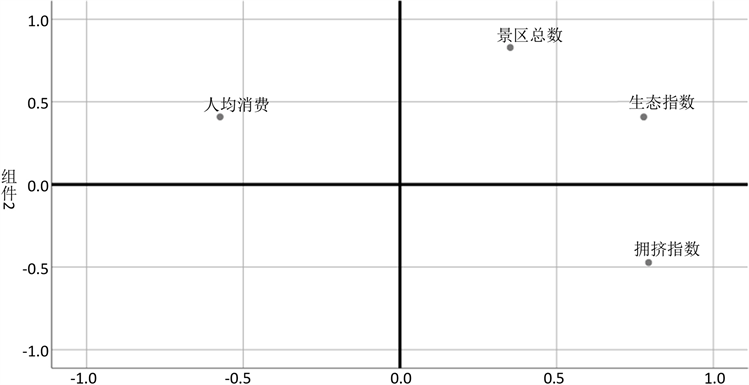Figure 1. Component diagramTable 3. Component matrix a after rotation

a旋转在3次迭代后已收敛。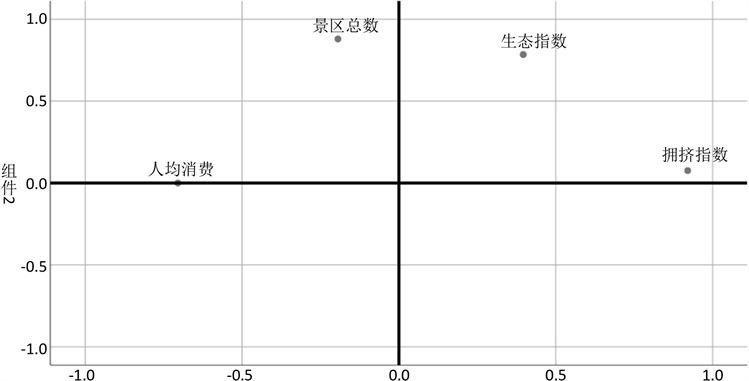Figure 2. Component diagram after rotation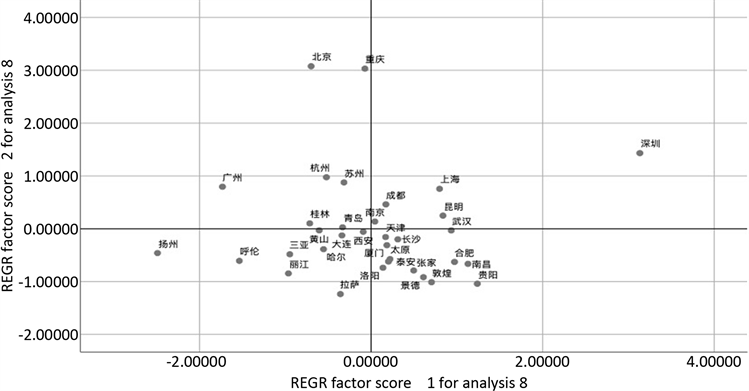Figure 3. Scatter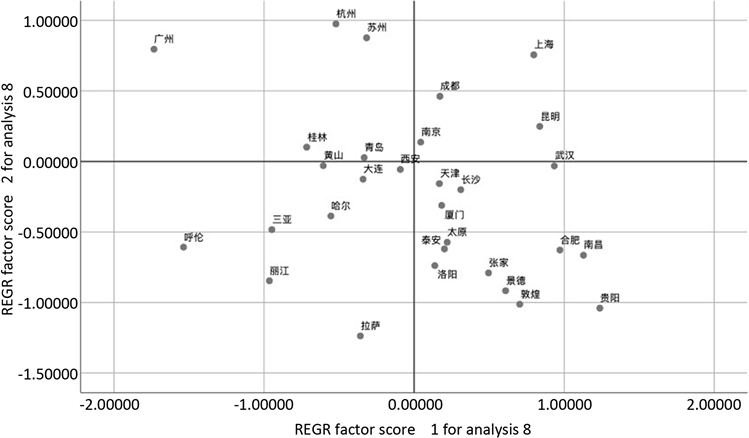Figure 4. Scatter plot after eliminating variation points

4.2. 聚类分析

5. 结论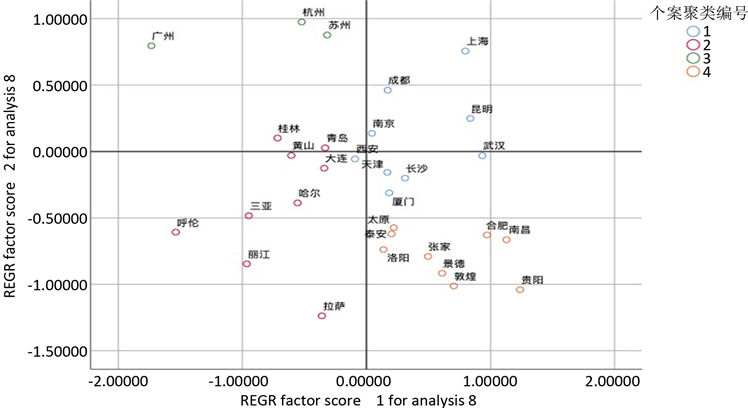Figure 5. Cluster analysis chart

1) 在主成分1和主成分2的构建中，主成分1代价指数和主成分2硬件指数比较客观地反映城市旅游的现状，两个主成分是符合实际的。

2) 在对各个城市的描述中，我们所构造的评判指数是行之有效的。在这一条件下提取出的两个主成分(硬件指数、代价指数)对各个城市的描述有良好的区分度和一定的指导意义。通过对城市的评价变量构造和处理，能够在一定范围内进行一定程度上的对比。

3) 发现一些城市如北京、深圳等在旅游的某一指数远高于其他城市，说明此城市在旅游中担当着领头羊的作用，对于旅游发展有重要影响。

  倪向丽. 我国省际旅游产业竞争力因子聚类评估[J]. 统计与决策, 2018, 34(21): 54-56.  张洪, 吕倩. “一带一路”沿线省份旅游效率演进研究[J]. 统计与决策, 2018, 34(16): 61-65.  邹泉. 基于投入产出模型的中国各省旅游竞争力研究[J]. 国土与自然资源研究, 2018(4): 51-58.  付志伟. 基于SPSS分析的浙江省区域旅游比较研究[D]: [硕士学位论文]. 杭州: 浙江大学, 2011.  俞彤. 基于SPSS的广东省城市旅游竞争力比较研究[J]. 四川旅游学院学报, 2016(4): 45-49.  李创新, 马耀峰, 高军. 基于SPSS的中国各省区旅游竞争力研究[J]. 软科学, 2018, 22(4): 98-104.  刘欣. 基于SPSS的省际旅游业综合竞争力评价分析——以河北省为例[J]. 北方经贸, 2015(8): 307-308.  朱星宇, 陈勇强. SPSS多元统计分析方法及应用[M]. 北京: 清华大学出版社, 2011.  李帅旗. 基于主成分分析的皖江城市带城市竞争力评价研究[D]: [硕士学位论文]. 重庆: 重庆交通大学, 2015.  王珂, 王雅文, 朱家明. 基于主成分分析的皖江城市带绿色发展水平的评价[J]. 哈尔滨师范大学自然科学学报, 2018, 34(2): 52-57.  王璐. 基于主成分分析的滇中城市群城市竞争力评价研究[J]. 经济视野, 2014(10): 1.  徐哲, 等. 应用统计学: 经济与管理的数据分析[M]. 北京: 清华大学出版社, 2015.